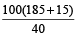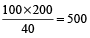### Percentage: Formulas, Tricks, Examples

Percentage is a way of expressing a number, especially a ratio, as a fraction of 100. The word is derived from the Latin per centum meaning ‘by the hundred’. It is often denoted using the percent sign, ‘%’, or the abbreviation ‘pct.’ For example, 35% (read as ‘thirty-five percent’) is equal to 35/100, or 0.35.

### Quicker Methods to Solve the Problems

For converting a fraction or a decimal to a Percentage, multiply it by hundred

Example 1: Convert the fraction 3/5 into percent fraction.

Solution: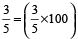% = 60%

Example 2: Convert the fraction 3.5/100 into percent fraction

Solution: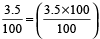% = 3.5%

For converting a percentage to a fraction or decimal, divide by hundred.

Example 3: What is the fraction of 60%?

Solution: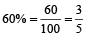If price of a commodity is increased by x%, the consumption should be reduced, so that the expense remains the same, by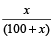× 100%.

Example 4: If the price of sugar is increased by 25%, find how much percent a family must reduce their consumption of sugar so as not to increase the expenditure of the family?

Solution: Reduction in consumption of sugar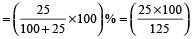% = 20%

If A is x % greater than B, then B will be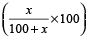% lesser than A.

Example 5: If the price of Kerosene oil falls by 10%, find how much perecent can a householder increase its consumption, so as not to decrease expenditure on this item?

Solution: Increase in consumption of Kerosene oil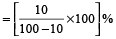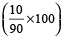% = 11.11%

If price of a commodity is decreased by x %, the consumption can be increased, so that the expense remains the same, by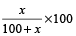%

Example 6: If Ravi’s salary is 50% more than that of Gopal’s, then how much percent is Gopal’s salary less than that of Ravi’s salary?

Solution: Gopal’s salary is less than that of Ravi’s by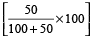% =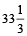%

If A is x % greater than B, then B will be% lesser than A.

Example 7: If income of Rekha is 30% less than that of Vina, then how much percent is Vina’s income more than that of Rekha?

Solution: Vina’s income is more than that of Rekha by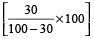%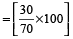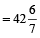%

### Population Formula

If the original population of a town is P, and the annual increase is r %, then the population after n years is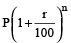and population before n years =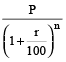If the annual decrease be r %, then the population after n years is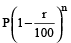and population before n years =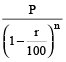Example 8: The population of a certain town increased at a certain rate per cent per annum. Now it is 456976. Four years ago, it was 390625. What will it be 2 years hence?

Solution: Suppose the population increases at r% per annum.

Then,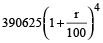= 456976

∴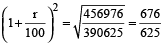Population 2 years hence = 456976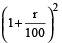= 456976 ×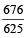= 494265 approximately.

### First Increase and then decrease

If the value is first increased by x % and then decreased by y% then there is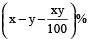increase or decrease, according to the +ve or –ve sign respectively.

Example 9: A number is increased by 10%. and then it is decreased by 10%. Find the net increase or decrease per cent.

Solution: % change =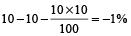i.e., 1% decrease.

Average percentage rate of change over a period.×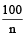% [where n = period]

The percentage error =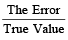× 100%

### Successive Increase or decrease

If the value is increased successively by x % and y % then the final increase is given by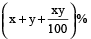If the value is decreased successively by x % and y % then the final decrease is given by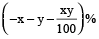Example 10: The price of a car is decreased by 10 % and 20 % in two successive years. What per cent of price of a car is decreased after two years?

Solution: Put x = – 10 and y = – 20, then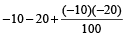= – 28%

∴ The price of the car decreases by 28%.

### Student and Marks

The percentage of passing marks in an examination is x%. If a candidate who scores y marks fails by z marks, then the maximum marks

M =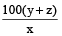A candidate scoring x % in an examination fails by ‘a’ marks, while another candidate who scores y% marks gets ‘b’ marks more than the minimum required passing marks. Then the maximum marks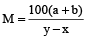.

Example 11: Vishal requires 40% to pass. If he gets 185 marks, falls short by 15 marks, what was the maximum he could have got?

Solution: If Vishal has 15 marks more, he could have scored 40% marks.

Now, 15 marks more than 185 is 185 + 15 = 200

Let the maximum marks be x, then 40% of x = 200

or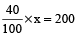or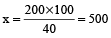Thus, maximum marks = 500

Quicker method:

Maximum marks

=